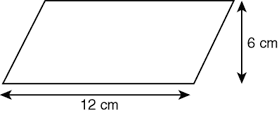Przejdź do głównych treści Przejdź do pola wyszukiwania

Definition: parallelogram from The Penguin Dictionary of Science

A ➤quadrilateral with two pairs of parallel sides.

Summary Article: parallelogram
From The Hutchinson Unabridged Encyclopedia with Atlas and Weather Guide

A quadrilateral (a shape with four sides) with opposite pairs of sides equal in length and parallel, and opposite angles equal. The diagonals of a parallelogram bisect each other. Its area is the product of the length (l) of one side and the perpendicular distance (height h) between this and the opposite side; the formula is A = l × h. In the special case when all four sides are equal in length, the parallelogram is known as a rhombus, and when the internal angles are right angles, it is a rectangle or square.

For example, to find the area of a parallelogram 12 cm long and 6 cm high:A = l × h, so

A = 12 × 6 = 72 cm2

images

parallelogram© RM, 2018. All rights reserved.

Pokrewne wpisy w Credo

Full text Article rectangle
Philip's Encyclopedia

Four-sided geometric figure (quadrilateral), the interior angles of which are right angles and each pair of opposite sides is of equal length...

Full text Article rectangle
The Hutchinson Unabridged Encyclopedia with Atlas and Weather Guide

Quadrilateral (four-sided plane figure) with opposite sides equal and parallel and with each interior angle a right angle (90°). Its area A is the pr

Full text Article rectangle
The Penguin Dictionary of Mathematics

A quadrilateral with all four angles right angles. The pairs of opposite sides are equal. If all four sides are equal, the rectangle is a...

Patrz więcej wyników Credo